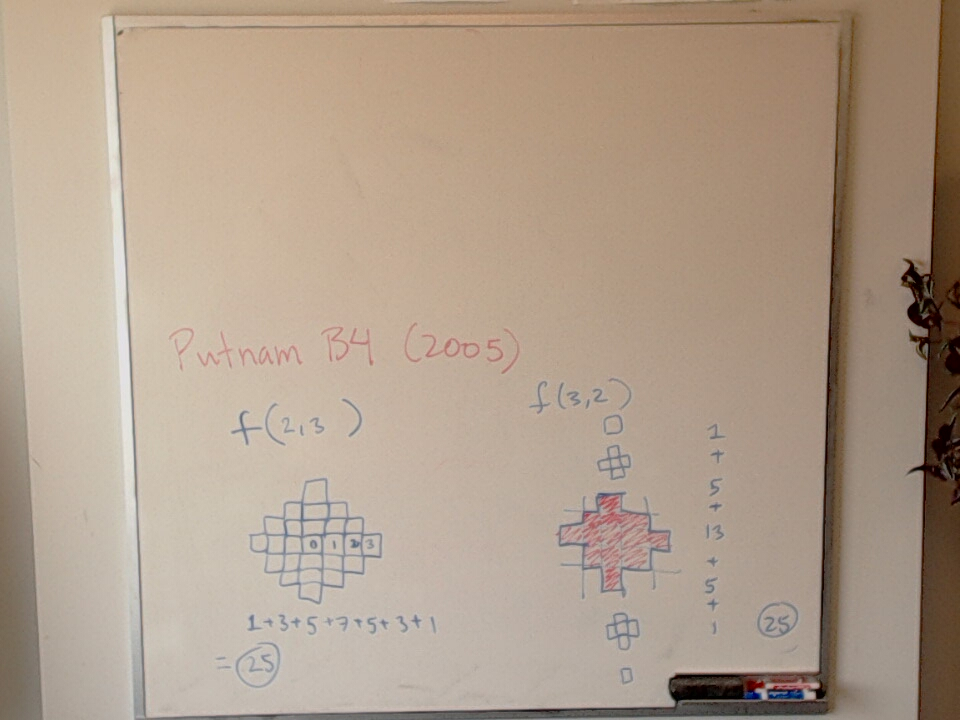# Putnam B4 2005

Jun 19, 2023For positive integers $m$ and $n$, let $f(m, n)$ denote the number of $n$-tuples $(x_1, x_2, \dots , x_n)$ of integers such that $|x_1|+|x_2|+ \cdots +|x_n| \leq m$. Show that $f(m, n) = f(n, m)$.

The photo on the blackboard verifies that $f(2,3) = f(3,2)$.

Related tags: